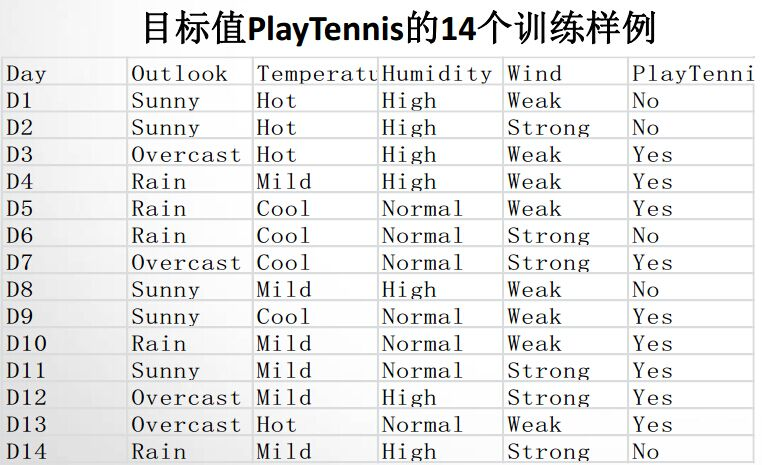# 一个例子# 信息熵

H(X)=i=1np(xi)logp(xi)$H(X)=-\sum\limits_{i=1} ^{n} p(x_i)\log p(x_i)$

p(y=yes)=514$p(y=yes)=\frac{5}{14}$

p(y=no)=914$p(y=no)=\frac{9}{14}$

# 条件熵

H(Y|X)=xXp(x)H(Y|X=x)$H(Y|X)=\sum\limits_{x\in X}p(x)H(Y|X=x)$

p(x=normal)=7/14=1/2=p2

H(Y|X=high)=-4/7*log(4/7)-3/7*log(3/7) = 0.6829
H(Y|X=normal)=-1/7*log(1/7)-6/7*log(6/7) = 0.4101

# 信息增益©️2019 CSDN 皮肤主题: 大白 设计师: CSDN官方博客